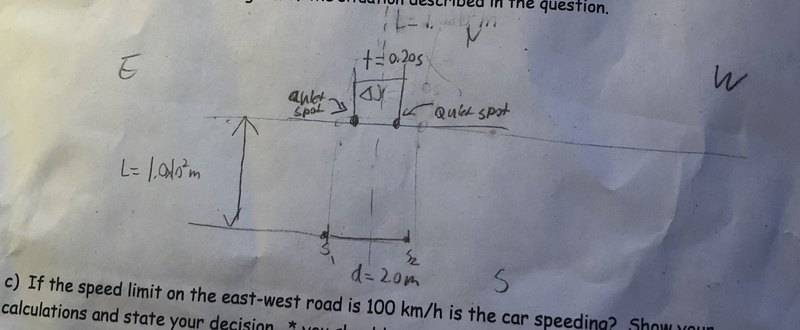## Homework Statement

[Answer is V = 25m/s, however, how do I get that answer? Thank you!] A police cruiser sets up a novel radar speed trap, consisting of two transmitting antennas at the edge of a main north-south road. One antenna is 2.0 m [W] of the other. The antennas, essentially point sources of continuous radio waves, are fed from a common transmitter with a frequency of 9 3.0 10 ^9 Hz . The trap is set for cars travelling south. A motorist drive a car equipped with a “radar detector” along an east-west road that crosses the main road at a level intersection 100 m [N] of the radar trap. The motorist hears a series of beeps upon driving west through the intersection. The time interval between succesive quiet spots is 0.2s as the car crosses the main road. How fast is the car moving?

## The Attempt at a Solution

Unfortunately I cannot figure out a way to get V = 25 m/s, however that is the answer. It seems as though there are too many unknowns. Any help would be appreciated, thank you.

berkeman
Mentor
Welcome to the PF.Can you post a diagram of the problem? If none was supplied, you can just upload a PDF or JPEG copy of the sketch that you have made.

What are the Relevant Equations (that's #2 on the Template -- it looks like you deleted that section).

What causes "Beeps" in this problem?

•C F
gneill
Mentor
Hi C F,

Welcome to Physics Forums!

Can you show us what you have tried? We need to see where you're going off track, and the forum rules don't allow helpers to chime in until they've got something from you to work with.

•C F
Hello sir. I've spent literally 4 hours trying to solve this.
Basically the given variables are

wavelength = ?
velocity = ?
delta x (nodal line distance) = ?
f = 3.0 * 10^9 Hz
d = 2.0 m
L = 1.0 * 10^2 m
t = 0.20 s

The equations that are relevant are
v = f * wavelength (universal wave equations)
delta x = t * v
delta x / L = wavelength /d

The beeps represent constructive interference and the quiet sports represent nodal lines (destructive interference).

I drew a diagram and know from the question that delta x (distance) is 0.2 s. So if I knew the velocity then I could get delta x. Hence the reason why I wrote the equation.

To approach the question what I did was to actually make system of equations but then noticed that the unknown variables just cancel out on each other and cannot be solved.
The system of equations will be three unknowns with two unknowns on each. However since there is no addition and it's just a pure relationship it is holding me back.

I drew a diagram#### Attachments

gneill
Mentor
Are you sure that the problem statement is exactly as it was give to you? No typos?

At 93 GHz frequency I'd think that the fringe spacing would be pretty short, less than a meter anyways at 100 m distance from the sources. If the correct answer is v = 25 m/s, then with a Δt between quiet zones of 0.2 s, the distance between the zones should be

##\Delta x = v \Delta t = 5 m##.

I don't see how to get that with the given values.

Although the distance between the sources is not terribly much smaller than the distance to the car, a rough approximation of the distance between fringes can be found as

##\Delta x \approx D \frac{\lambda}{d}## which gives ##\Delta x \approx 0.161\;m##.

How did you know the wave length (λ) when you said

"Δ x ≈ D λ / d which gives Δ x ≈ 0.161 m Δx≈0.161m." ?

I have been trying to solve for it.

Also why is the frequency 93 GHz when it is 3.0 * 10^9 Hz?

Thank you.

I want to know how to tweak with the relationships to get any of the unknown values. Could you please show me how?

gneill
Mentor
How did you know the wave length (λ) when you said
Also why is the frequency 93 GHz when it is 3.0 * 10^9 Hz?
...are fed from a common transmitter with a frequency of 9 3.0 10 ^9 Hz .
I assumed that the space between the 9 and 3 was a typo. That's why I asked about the problem statement being a faithful rendition of the original. The wavelength then became:

##\lambda = \frac{c}{f}##

This new information makes a lot of difference! Now we find:

##\Delta x \approx D \frac{\lambda}{d}## so that ##\Delta x \approx 4.997\;m## or near enough to 5 m as expected from the given answer.

I want to know how to tweak with the relationships to get any of the unknown values. Could you please show me how?
We can't do it for you, only guide your own attempts that you show us. I can suggest that you look at web sites that show how interference works for the double slit experiment (this is just a fancy version of that). The Wikipedia entry in its Classical wave-optics formulation section might be helpful. It actually shows the same approximations that I used.

•berkeman